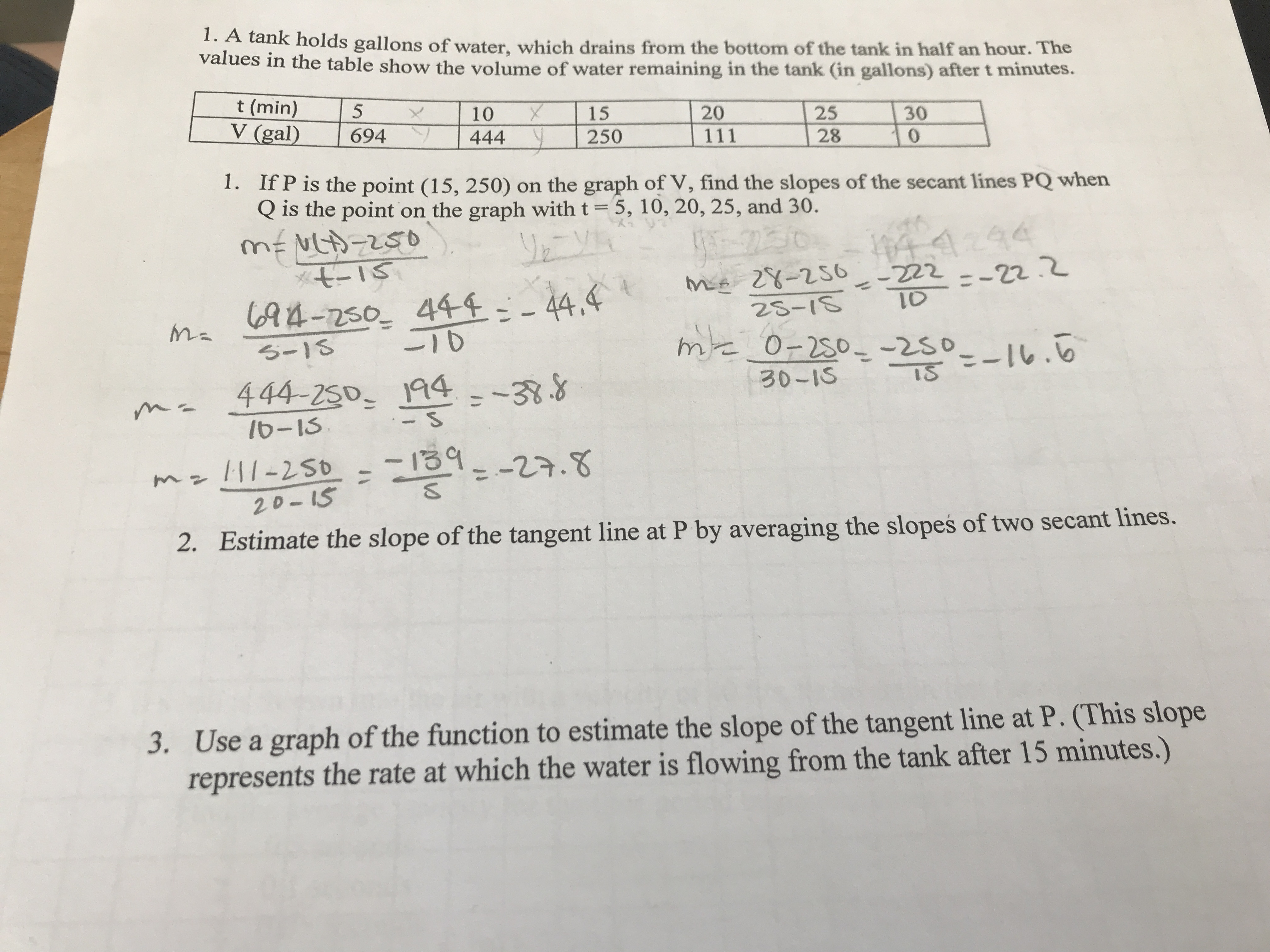# 1. A tank holds gallons of water, which drains from the bottom of the tank in half an hour. Thevalues in the table show the volume of water remaining in the tank (in gallons) after t minutes.t (min)V (gal)1015202530694444250111280.1. If P is the point (15, 250) on the graph of V, find the slopes of the secant lines PQ whenQ is the point on the graph with t= 5, 10, 20, 25, and 30.Lカ-250メモーIS694-250 444=-44.4る-134294me 28-25625-18-222--22.%3D44,410-10me O-250--2so_-16.6444-250 194--38.830-1S16-13mz!ll-250 -139|1-250%3D1=-27.820-152. Estimate the slope of the tangent line at P by averaging the slopes of two secant lines.3. Use a graph of the function to estimate the slope of the tangent line at P. (This sloperepresents the rate at which the water is flowing from the tank after 15 minutes.)

Question
24 views

3) use a graph of the function to estimate the slope of the tangent line at P. (This slope represents the rate at which the water is flowing from the tank after 15 minutes)help_outlineImage Transcriptionclose1. A tank holds gallons of water, which drains from the bottom of the tank in half an hour. The values in the table show the volume of water remaining in the tank (in gallons) after t minutes. t (min) V (gal) 10 15 20 25 30 694 444 250 111 28 0. 1. If P is the point (15, 250) on the graph of V, find the slopes of the secant lines PQ when Q is the point on the graph with t= 5, 10, 20, 25, and 30. Lカ-250 メモーIS 694-250 444=-44.4 る-13 4294 me 28-256 25-18 -222--22. %3D 44,4 10 -10 me O-250--2so_-16.6 444-250 194--38.8 30-1S 16-13 mz!ll-250 -139 |1-250 %3D 1=-27.8 20-15 2. Estimate the slope of the tangent line at P by averaging the slopes of two secant lines. 3. Use a graph of the function to estimate the slope of the tangent line at P. (This slope represents the rate at which the water is flowing from the tank after 15 minutes.) fullscreen
check_circle

Step 1

Draw a graph by plotting the points (5, 694), (10, 444), (15, 250), (20, 111), (25, 28) and (30, 0). Draw the slope of tangent line at P.

Step 2

Then we ha...

### Want to see the full answer?

See Solution

#### Want to see this answer and more?

Solutions are written by subject experts who are available 24/7. Questions are typically answered within 1 hour.*

See Solution
*Response times may vary by subject and question.
Tagged in

### Calculus